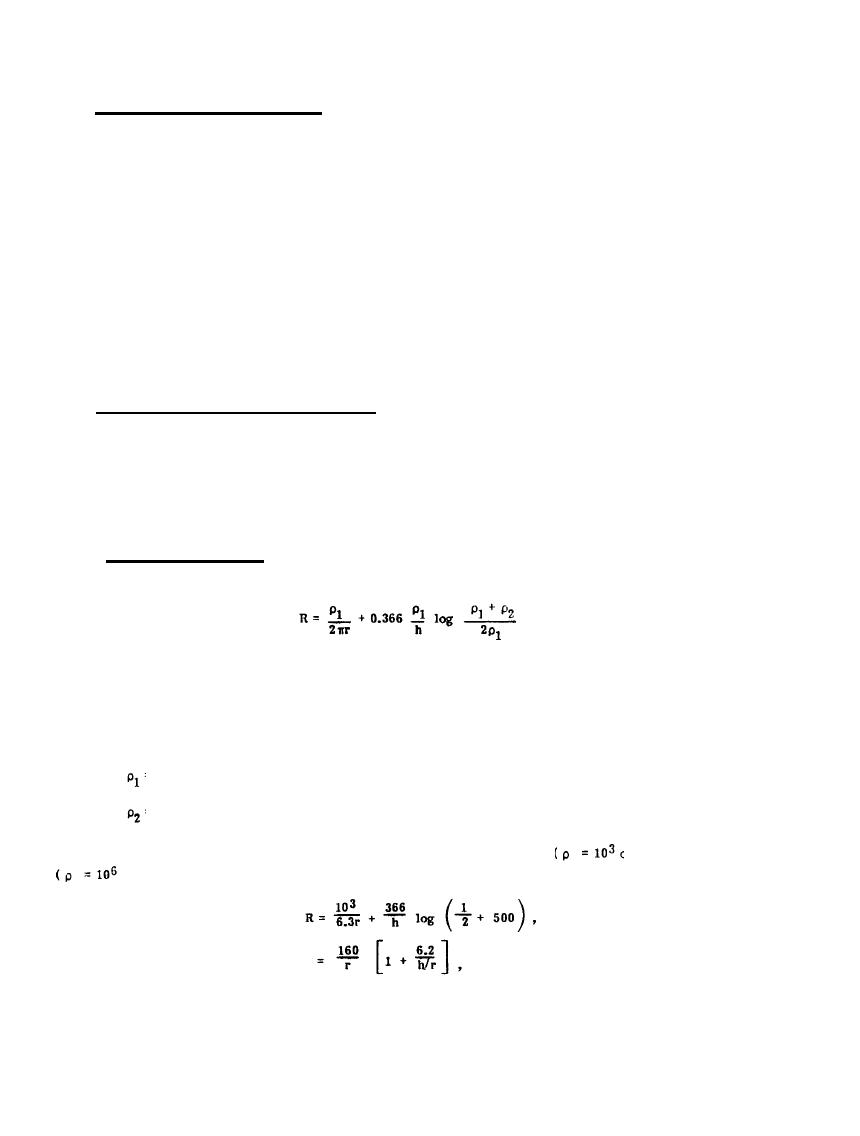Transient Impedance of ElectrodesCustom SearchMIL-HDBK-419A
2.6.3 Transient Impedance of Electrodes. The expressions given for electrode resistance assume perfect
conductivity for the conductors of an electrode.  Such an assumption introduces very little error in the
calculation of the electrode dc resistance, but if the electrode must dissipate the impulsive energy of a
lightning stroke, its impedance as a function of time must be considered.  When a single star electrode,
containing 305 meters (1000 feet) of conductor, is subjected to a surge of lightning current, the initial value of
its effective impedance is about ten times the dc resistance (2-11). This initial value is termed the surge
As the wave of energy propagates through the electrode system, more and more of the wire of the
electrode makes effective contact between the propagating energy and the medium which dissipates the energy.
It is clear that a given length of wire will couple lightning energy more efficiently into the earth if the
electrode is in the form of a star than if it were a single conductor. This is illustrated in Figure 2-13 where it
is indicated that as the energy surges down an electrode (at a velocity in the neighborhood of 100 meters (333
feet) per microsecond), the transient impedance of the electrode decreases and approaches the dc resistance
value.
2.6.4 Effects of Nonhomogeneous (Layered) Earth. The previous derivations assumed homogeneous earth. A
qualitative understanding of the effects of non-uniform earth resistivity can be deduced from Figure 2-14
which illustrates the electric equipotential surfaces and current flow in layered earth when the earthing
electrode is a small hemisphere. The lines radiating outward from the earth electrode indicate the flow of
current. Not surprisingly, if the resistivity of the deeper layer is high, relative to the upper layer, nearly all of
the current is confined to the upper layer of earth.
2.6.4.1 Hemispherical Electrode. An approximate expression (2-3) for the resistance to earth of a small
hemispherical electrode in layered earth is
(2-32)
where
r = hemisphere radius (assumed less than h),
h = thickness of superficial layer,
= resistivity of superficial layer,
= resistivity of deep layer.
An interesting example is the case of a superficial layer of low resistivity soil
ohm-cm) over granite
shin-cm):
(2-33)
2-32Integrated Publishing, Inc.